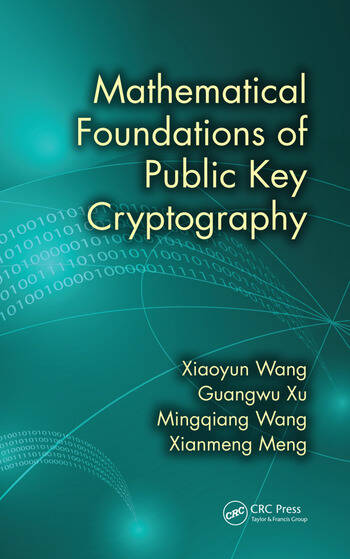# Mathematical Foundations of Public Key Cryptography

## 1st Edition

Xiaoyun Wang, Guangwu Xu, Mingqiang Wang, Xianmeng Meng

CRC Press
Published October 21, 2015
Reference - 236 Pages
ISBN 9781498702232 - CAT# K24602

For Instructors Request Inspection Copy

was \$86.95

USD\$69.56

SAVE ~\$17.39

FREE Standard Shipping!

## Preview

### Summary

In Mathematical Foundations of Public Key Cryptography, the authors integrate the results of more than 20 years of research and teaching experience to help students bridge the gap between math theory and crypto practice. The book provides a theoretical structure of fundamental number theory and algebra knowledge supporting public-key cryptography.

Rather than simply combining number theory and modern algebra, this textbook features the interdisciplinary characteristics of cryptography—revealing the integrations of mathematical theories and public-key cryptographic applications. Incorporating the complexity theory of algorithms throughout, it introduces the basic number theoretic and algebraic algorithms and their complexities to provide a preliminary understanding of the applications of mathematical theories in cryptographic algorithms.

Supplying a seamless integration of cryptography and mathematics, the book includes coverage of elementary number theory; algebraic structure and attributes of group, ring, and field; cryptography-related computing complexity and basic algorithms, as well as lattice and fundamental methods of lattice cryptanalysis.

The text consists of 11 chapters. Basic theory and tools of elementary number theory, such as congruences, primitive roots, residue classes, and continued fractions, are covered in Chapters 1-6. The basic concepts of abstract algebra are introduced in Chapters 7-9, where three basic algebraic structures of groups, rings, and fields and their properties are explained.

Chapter 10 is about computational complexities of several related mathematical algorithms, and hard problems such as integer factorization and discrete logarithm. Chapter 11 presents the basics of lattice theory and the lattice basis reduction algorithm—the LLL algorithm and its application in the cryptanalysis of the RSA algorithm.

Containing a number of exercises on key algorithms, the book is suitable for use as a textbook for undergraduate students and first-year graduate students in information security programs. It is also an ideal reference book for cryptography professionals looking to master public-key cryptography.

#### Instructors

We provide complimentary e-inspection copies of primary textbooks to instructors considering our books for course adoption.